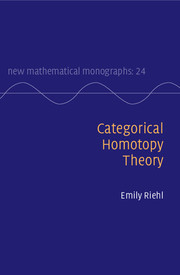## Categorical Homotopy TheoryLecture notes from a graduate topics topics course, entitled Categorical Homotopy Theory, taught in the Spring of 2012 at Harvard, were published in May 2014 by Cambridge University Press.

Thanks to a special arrangement with Cambridge University Press, I am also able to host a free PDF copy, which can be found here. This material has been published by Cambridge University Press as Categorical Homotopy Theory by Emily Riehl. This version is free to view and download for personal use only. Not for re-distribution, re-sale or use in derivative works. © Emily Riehl 2014.

CONTENTS

A brief description of the contents can be found here.

ERRATA

I'm grateful to those who have written to point out the following mistakes. Please get in touch if you think you may have found another error. The page numbers refer to the published version.

• [page 49, Definitions 3.7.2-3] The isomorphism in 3.7.2 should be V-natural in n, while the isomorphism in 3.7.3 should be V-natural in m. See here for a discussion of this point. Alternatively, Remark 3.7.4 can be taken as a definition of what it means for a V-category to be tensored and/or cotensored.
• [page 65, proof of Lemma 4.4.2] There is nothing technically incorrect but the notation is very misleading. Because Σ : el(ND)op → Δop, the objects in the comma category Σ/[n] are pairs given by a composable sequence of arrows d0→...→dm together with a morphism [m] → [n] in Δop. More directly, if we instead consider Σ as a functor Σ: el(ND) → Δ then the comma category appearing in the text is the opposite of the comma category [n]/Σ.

To see that [n]/Σ decomposes as a disjoint union of categories with an initial object, consider a general object [n] → Σ(d0→...→dm). By the Eilenberg-Zilber lemma, the map [n] → [m] factors uniquely as an epimorphism [n] → [k] followed by a monomorphism [k] → [m]. The initial object in this component is formed by taking the subsequence of k composable arrows identified by the monomorphism [k] → [m], then inserting identities as specified by the epimorphism [n] → [k], and then using the resulting sequence of n composable arrows to define an object in [n]/Σ whose morphism cmponent is the identity at [n]. This then proves the claim made in the text, that the opposite of this category decomposes as a disjoint union of categories with a terminal object.

• [page 107, Remark 7.2.10] The category C§ introduced by Mac Lane is for computing ends as ordinary limits; to compute coends as colimits, you need to use the opposite category. Taking Mac Lane's definition, the obvious functor C§ → twC is initial (not final).
• [page 111, Definition 9.1.5] The enriched bar and cobar constructions are defined relative to a cosimplicial object in V. Thus, the domain of the functor Δ should not have an “op”, a typo that appears in the first sentence of Definition 9.1.5 and also in the sentence preceding the definition.
• [page 129, Example 8.3.9] As remarked above, the functor C§ → twC is initial (not final). A final functor, relevant for computing coends as ordinary colimits, is given by replacing both categories by their opposites.
• [page 195, proof of Theorem 12.2.2] In the first displayed diagram, the index of the interior coproduct should be Sq(j,Rnf), not Sq(j,f).
• [page 299, after Theorem 17.1.1] The category of finite ordinals and order-preserving maps is strictly monoidal, with the monoidal product given by ordinal sum, but it is not symmetric monoidal. On objects, we have [n] + [m] = [n+m+1] = [m] + [n], but this (identity) isomorphism is not natural.
• [page 302, end of section 17.1] The proof of Theorem 17.1.1 reveals that you can solve lifting problems between outer horn inclusions and inner fibrations between quasi-categories, provided the image of the initial or terminal edge of the horn, respectively, is an isomorphism. More discussion on a closely related question may be found here.
CATEGORICAL PREREQUISITES

This brief survey of categorical concepts intends to convey the philosophy and terminology of category theory by exploring its implications in elementary examples.